Figure 2.1 A scatterplot produced by the plot() function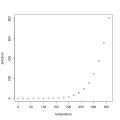R code
 Figure 2.2 Four variations on a scatterplot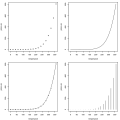R code
 Figure 2.3 Plotting an "lm" object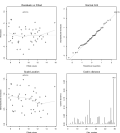R code
 Figure 2.4 Plotting an agnes object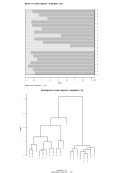R code
 Figure 2.5 Plots for a single variable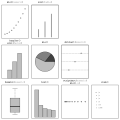R code
 Figure 2.6 Plots of two variables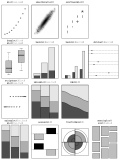R code
 Figure 2.7 Plots of many variables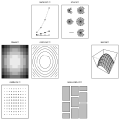R code
 Figure 2.8 Modifying default barplot() and boxplot() output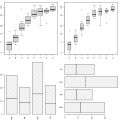R code
 Figure 2.9 Standard arguments for high-level functions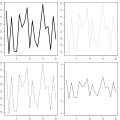R code
 Figure 2.10 Some specialized plots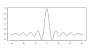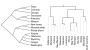R code